```       __                                             __
___ / /____ _  _____ ______ _____ ____    ___  ___ / /_
(_-</ __/ -_) |/ / -_) __/ // / _ `/ _ \_ / _ \/ -_) __/
/___/\__/\__/|___/\__/_/  \_, /\_,_/_//_(_)_//_/\__/\__/
/___/
```

### Weighted Stego-Image Explained

May 11, 2022

Weighted Stego-Image (WS) attempts to calculate the lengths of secret messages hidden in natural images by generating an estimate of the cover image and comparing it with a flipped version of the stego-image.

For simplicity, let us assume our cover image, c, is greyscale, 5 x 5 pixels, and each pixel is completely black with a value of 0:

0 0 0 0 0
0 0 0 0 0
0 0 0 0 0
0 0 0 0 0
0 0 0 0 0

If we use LSBR to sequentially embed the message 11100011 10001110 01100110 in c, this gives us the stego-image, s:

1 1 1 0 0
0 1 1 1 0
0 0 1 1 1
0 0 1 1 0
0 1 1 0 0

Typically half the LSBs in the cover image will already be set to the desired value. In the case of c, only 13 LSBs needed to be changed, even though the length of the message is 24 bits.

Next we create a new image called s-bar. This is simply s with all its LSBs flipped:

0 0 0 1 1
1 0 0 0 1
1 1 0 0 0
1 1 0 0 1
1 0 0 1 1

We create another image called s-alpha or the weighted stego-image. This image is created using the weighted average of every byte in s and s-bar. The formula for s-alpha is as follows:

s-alpha[i] = alpha * s[i] + (1 − alpha) * s-bar[i], where

* i = index of each byte in s and s-bar
* alpha = weight

Therefore if use an alpha of 0.5, the image produced using the above formula is as follows:

0.5 0.5 0.5 0.5 0.5
0.5 0.5 0.5 0.5 0.5
0.5 0.5 0.5 0.5 0.5
0.5 0.5 0.5 0.5 0.5
0.5 0.5 0.5 0.5 0.5

The euclidean distance between s-alpha and the cover image c is minimised when alpha = m/2n, where m is the number of bits in the hidden message, and n is the number of pixels in the image. Therefore if we can calculate this distance, we will be able to figure out m.

Calculating the euclidean distance between two images is the same as calculating the distance between two matrices. This is defined as follows: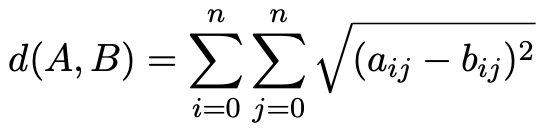Therefore the distance between s-alpha and c is as follows: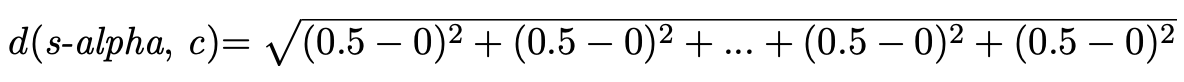In a real-world scenario we would not have access to c (we would only have access to s), so we cannot calculate the distance from s-alpha to c, however we can estimate the pixels of c using the weighted average of the pixels of s, and use this estimation in place of c. We do this by applying a weighted mask to s to produce f, the estimation of c.

Let us take a look at how a 3 x 3 mask can be used to estimate the first four pixels of c. We will use the following mask:

1 0 1
0 1 0
1 0 1

The first iteration is as follows: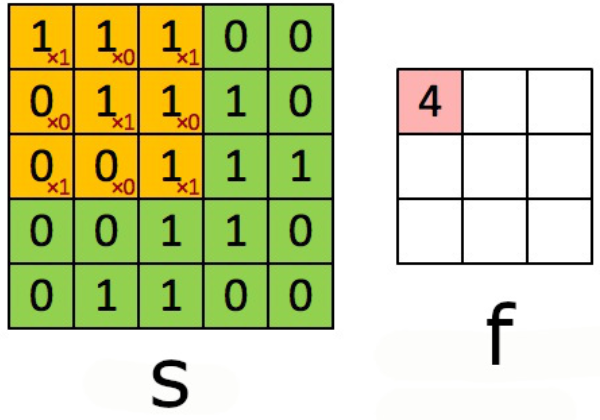The second iteration: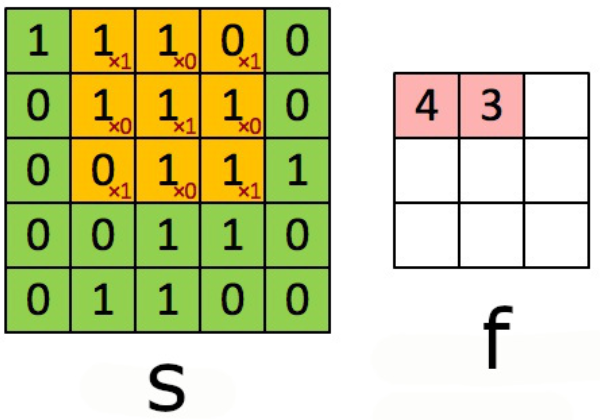The third iteration: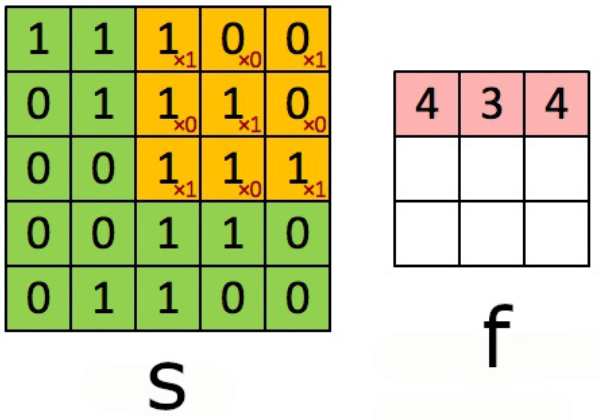The fourth iteration: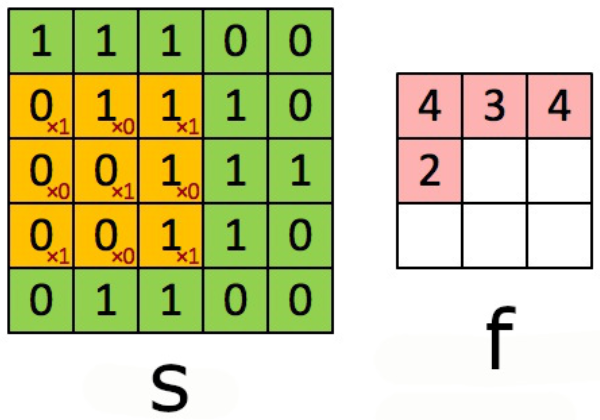After all iterations have completed, the estimation of c, f, is as follows:

4 3 4
2 4 3
2 3 4

Fridrich et al, the creators of WS, use a simple mask to estimate the value of each pixel. It consists of averaging four pixels surrounding each target pixel. Ker et al, who revisited WS, suggest a number of improvements to this including using unsymmetrical masks of varying sizes and weights. They suggest the following mask as optimal:

−0.25 0.5 −0.25
0.5  0    0.5
−0.25 0.5 −0.25

Using this optimal mask (with rounding) produces a better estimation of c:

0 2 0
1 1 1
1 1 0

As you can see, f is now very similar to c (more than 99% accurate), however it is 3 x 3 instead 5 x 5 due to the mask missing the edges and corners of c. This is usually a non-issue when using real-world images (usually much larger than 5 x 5 pixels) as the edges and corners only make up a small portion of the overall image.

Ker et al state the optimal mask might depend on which cover image is being analysed, so using weighted least-squares regression to determine the weights (a and b in the following mask) may be preferable. However they state this will double the computation time.

b a b
a 0 a
b a b

After determining the best estimate of c, we can find the distance between s-alpha and f, and solve for the alpha that minimises the distance between both images. As discussed above, the alpha that minimises the distance between both images should be around m/2n. We already have the value of n, so we just need to figure out m, the estimated length of the hidden message.

We know the distance between s-alpha and f is as follows: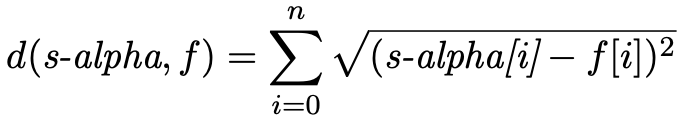where [i] is an iterator starting from the first byte in each image.

During the above calculation, the edges and corners of s-alpha are discarded to make it the same size as f.

Since we want the alpha that minimises the distance, the square root will not affect the minimisation (if the distance is minimal, the squared distance is also minimal), so we want the alpha that minimizes the sum of the square of the differences: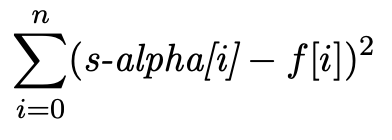If we take the derivative of this function and set it to zero, we can find where the slope of the function is also zero (the maxima and minima of the original function). This is the alpha which minimises the distance: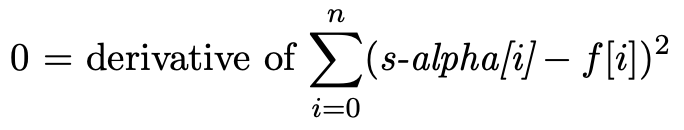We know s-alpha[i] is as follows:

s-alpha[i] = alpha * s[i] + (1 − alpha) * s-bar[i]

Therefore: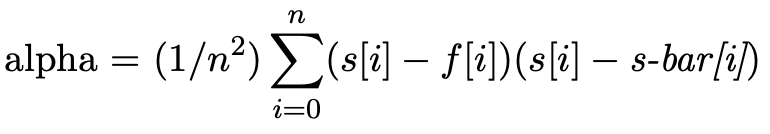Since the alpha is m/2n, we can calculate m, the length of the hidden message: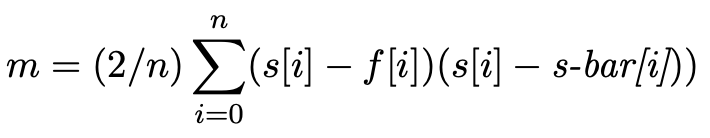To calculate m for an RGB image, each colour channel is treated like a separate greyscale-like image: one image using all the red bytes, one image using all the green bytes, and one image using all the blue bytes. The output is the estimation of LSB changes for each colour channel.